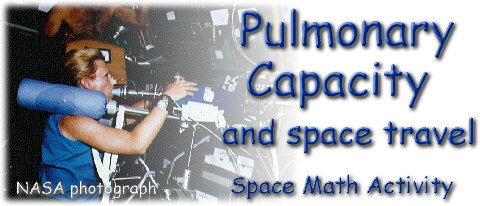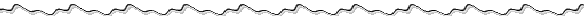NASA Project NOVA Activity Developer:  Donna Roberts

While we are on earth, gravity has a major effect upon how the air and blood in our lungs are distributed.  In space, the distribution of blood through the lungs becomes uniform and the heart, lungs and blood vessels no longer have to labor against gravity.

This activity will allow you to determine your breathing capacity while on the earth.

Activity:  To determine present breathing capacity

Mathematical Skills:  grade level 7-12, solving algebraic equations, substitution of known quantities, and conversions of units.

Materials:  String for measuring, ruler, round balloons, calculator

Directions:  This activity requires the assistance of a partner to help in measuring the circumference of each balloon.  Each balloon should be stretched several times before attempting to blow it up.  Inhale as much air as possible and exhale into the balloon.  The lungs should be emptied completely, but inhaling a second time is not permitted.

Measure the largest part of the balloon as the circumference using the string and the ruler.  Record information on the data sheet.Data Collection SheetStretch the balloon by blowing it up several  times before beginning the activity.

1.  Record the Circumference of balloon in CENTIMETERS:
 Trial #1 Trial #2 Trial #3 Trial #4 Trial #5 Trial #6

2.  Determine the Average Circumference from the 6 Trials.

3.  Using the formula for Circumference, and your average circumference, find the radius (Solve the circumference formula for r.)

4.  Calculate the VOLUME of the balloon (a sphere).  Your answer will be in cubic centimeters (cc).  This is your experimental lung capacity. Volume = ____________ cc (or ml)

5.  State your height in feet and inches: _____________

6.  State your height in total inches: _____________

7.  Convert height to centimeters: ___________  (1 inch = 2.54 centimeters)

8.  State your age in years: ________

 Theoretical Lung Capacity: V = 0.041 h - 0.018 a - 2.69 where V is vital lung capacity in liters,   h is height in centimeters,  and a is age in years. from American Lung Association

9
.  Determine your Theoretical Lung Capacity:   _________ liters

10.  Convert Theoretical Lung Capacity to milliliters.   Theoretical Capacity x 1000 = _________________ml

11.  Compare your experimental lung capacity to your theoretical lung capacity.   [If your experimental lung capacity is less than or equal to 1000 cc (l liter), there is a good chance that your breathing has a compromised capacity.]